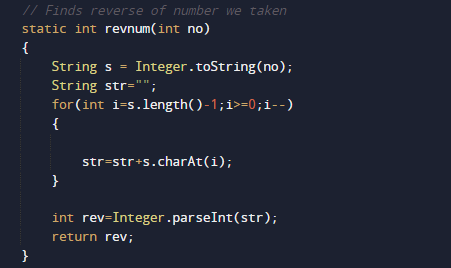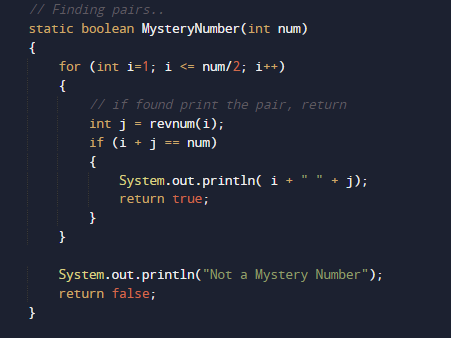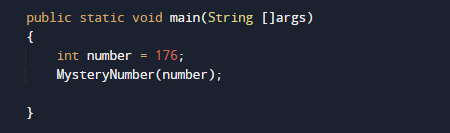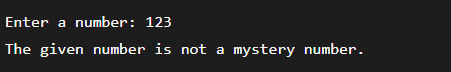# Program to check Mystery Number in Java

In this tutorial you will learn about the Program to check Mystery Number in Java and its application with practical example.

In this tutorial, we will learn to create a Java Program to check Mystery Number in Java using Java programming.

## Prerequisites

Before starting with this tutorial we assume that you are best aware of the following Java programming topics:

• Java Operators.
• Basic Input and Output function in Java.
• Class and Object in Java.
• Basic Java programming.
• If-else statements in Java.
• For loop in Java.
• User define functions.

### Mystery Number are Numbers

A mystery number is a number that lies between (22 to 198), i.e. 198>=N>=22.A number that can be expressed as the sum of two numbers and those two numbers should be the reverse of each other.

Example:  some the example of Mystery Number are:

132 = 93+39
154 = 68 + 86
176 = 79 + 97

## Program to check Mystery Number in Java

A number will be called a Mystery number if sum of two number and reverse of these two number must be same .We would first declared and initialized the required variables. Next, we would prompt user to input the value. Later we will find Mystery Number.

## Output

Mystery Number 176.In the above program, we have first declared and initialized a set variables required in the program.

## Find Mystery Number

• take a Number and initialize to number variable .
• Then Convert the number into String.
• And now reverse the String .
• Again, convert string to integer ,as shown in the image below.• Find the pair of numbers.• Compare the sum with the given number.If the sum and the given number are equal, the number is a mystery.Else its not a mystery number.We have implemented the above a Java program that checks if the given number is a mystery number or not.

In this tutorial we have learn about the Program to check Mystery Number in Java and its application with practical example. I hope you will like this tutorial.